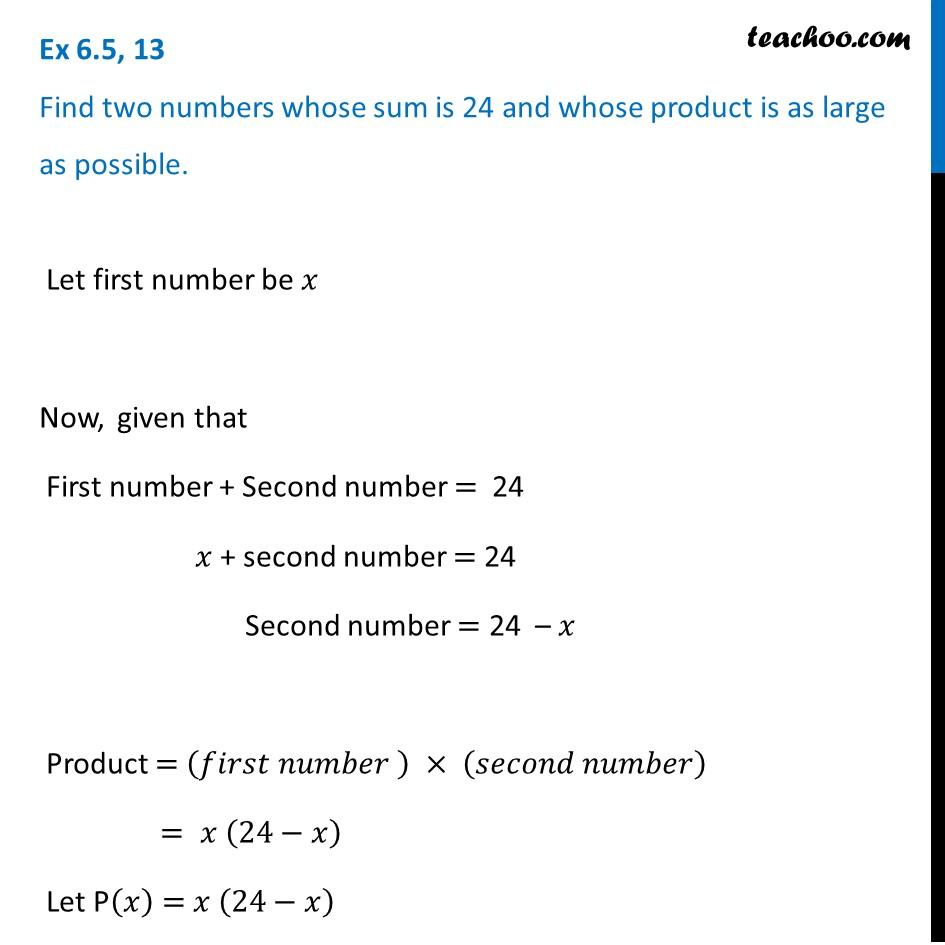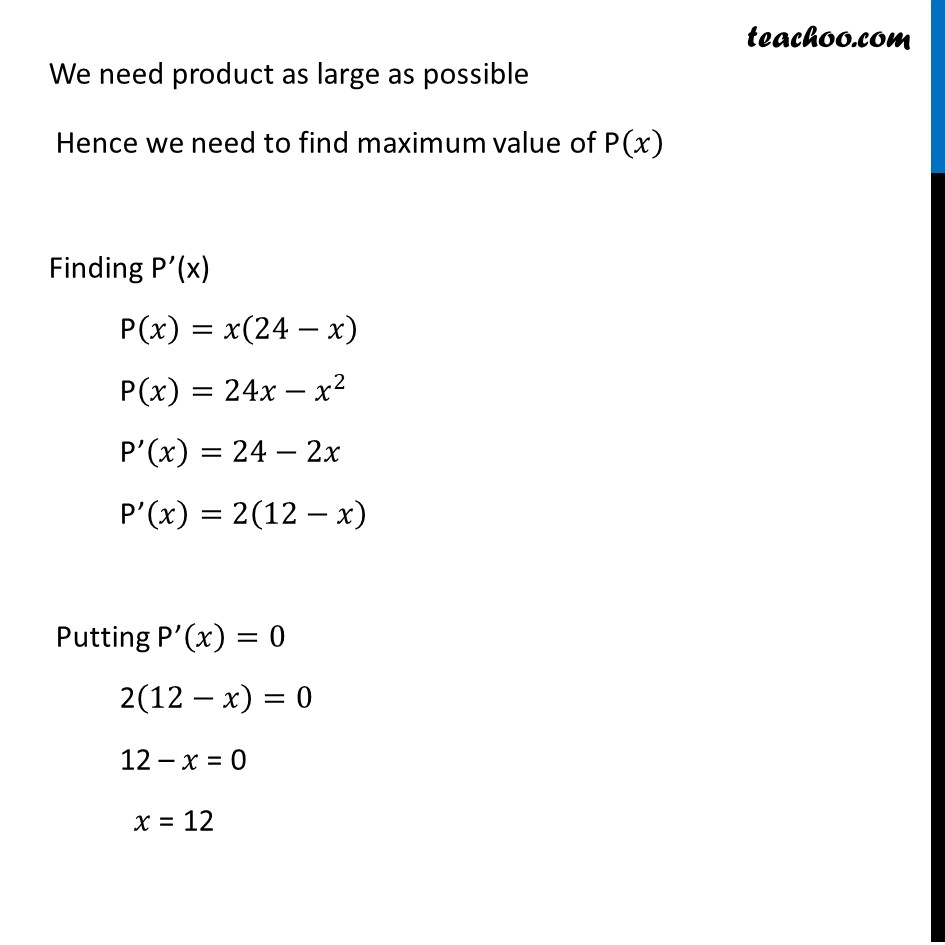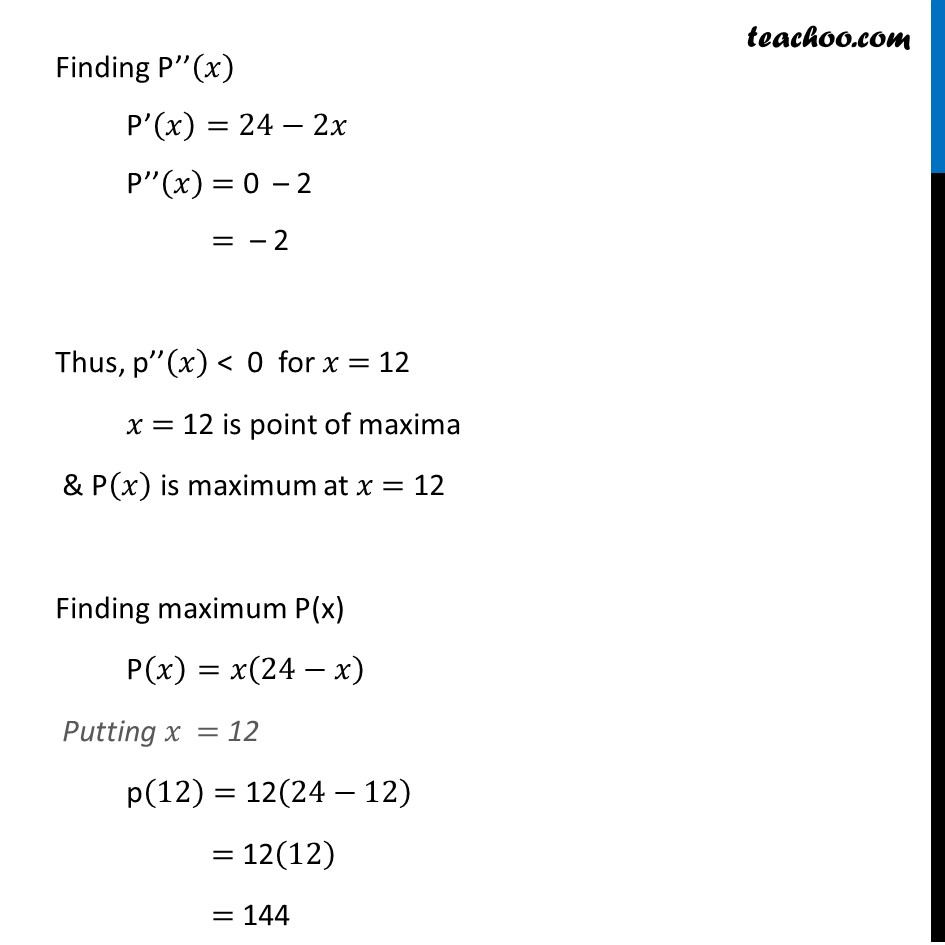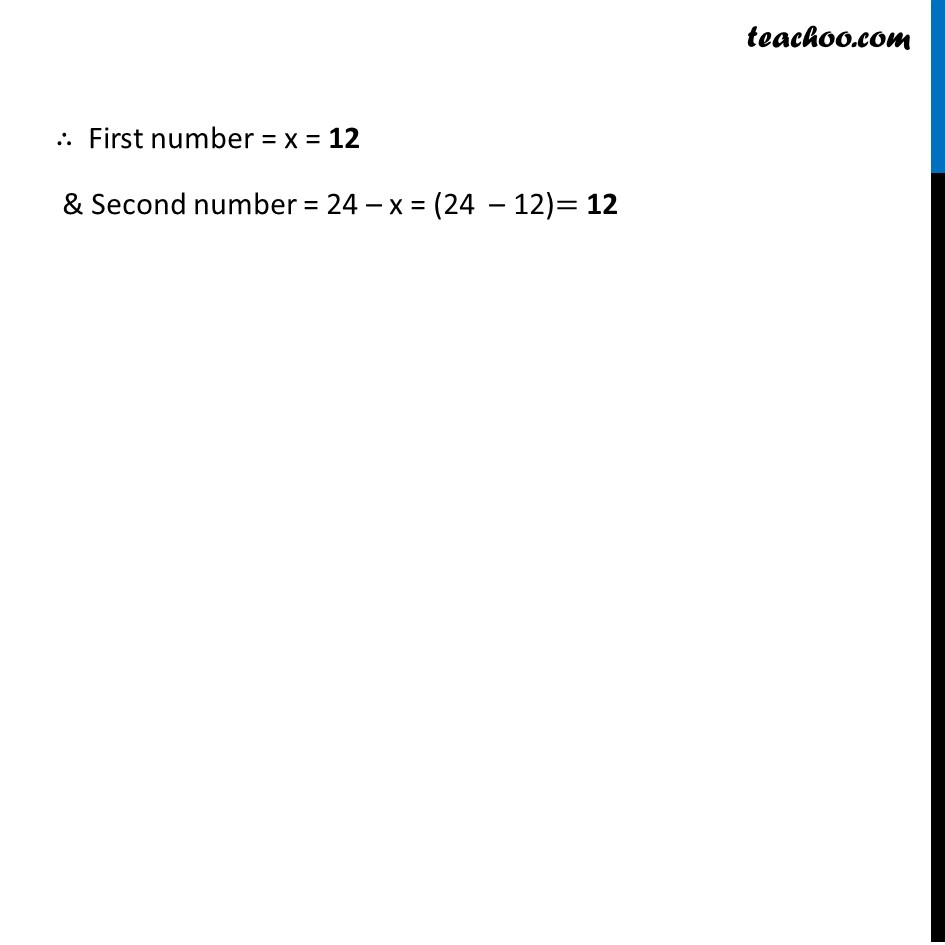Minima/ maxima (statement questions) - Number questions

Chapter 6 Class 12 Application of Derivatives
Concept wiseLearn in your speed, with individual attention - Teachoo Maths 1-on-1 Class

### Transcript

Ex 6.3, 13 Find two numbers whose sum is 24 and whose product is as large as possible. Let first number be 𝑥 Now, given that First number + Second number = 24 𝑥 + second number = 24 Second number = 24 – 𝑥 Product = (𝑓𝑖𝑟𝑠𝑡 𝑛𝑢𝑚𝑏𝑒𝑟 ) × (𝑠𝑒𝑐𝑜𝑛𝑑 𝑛𝑢𝑚𝑏𝑒𝑟) = 𝑥 (24−𝑥) Let P(𝑥) = 𝑥 (24−𝑥) We need product as large as possible Hence we need to find maximum value of P(𝑥) Finding P’(x) P(𝑥)=𝑥(24−𝑥) P(𝑥)=24𝑥−𝑥^2 P’(𝑥)=24−2𝑥 P’(𝑥)=2(12−𝑥) Putting P’(𝑥)=0 2(12−𝑥)=0 12 – 𝑥 = 0 𝑥 = 12 Finding P’’(𝑥) P’(𝑥)=24−2𝑥 P’’(𝑥) = 0 – 2 = – 2 Thus, p’’(𝑥) < 0 for 𝑥 = 12 𝑥 = 12 is point of maxima & P(𝑥) is maximum at 𝑥 = 12 Finding maximum P(x) P(𝑥)=𝑥(24−𝑥) Putting 𝑥 = 12 p(12)= 12(24−12) = 12(12) = 144 ∴ First number = x = 12 & Second number = 24 – x = (24 – 12)= 12